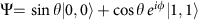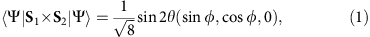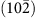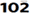# Ferroelectricity by Bose–Einstein condensation in a quantum magnet

## Abstract

The Bose–Einstein condensation is a fascinating phenomenon, which results from quantum statistics for identical particles with an integer spin. Surprising properties, such as superfluidity, vortex quantization or Josephson effect, appear owing to the macroscopic quantum coherence, which spontaneously develops in Bose–Einstein condensates. Realization of Bose–Einstein condensation is not restricted in fluids like liquid helium, a superconducting phase of paired electrons in a metal and laser-cooled dilute alkali atoms. Bosonic quasi-particles like exciton-polariton and magnon in solids-state systems can also undergo Bose–Einstein condensation in certain conditions. Here, we report that the quantum coherence in Bose–Einstein condensate of the magnon quasi particles yields spontaneous electric polarization in the quantum magnet TlCuCl3, leading to remarkable magnetoelectric effect. Very soft ferroelectricity is realized as a consequence of the O(2) symmetry breaking by magnon Bose–Einstein condensation. The finding of this ferroelectricity will open a new window to explore multi-functionality of quantum magnets.

## Introduction

TlCuCl3 is the first material whose field-induced quantum phase transition was classified as a realization of Bose–Einstein condensation (BEC) in the quantum magnets1. In this material, antiferromagnetic spin dimers, composed of a pair of Cu2+ ions with spin S=1/2, are three-dimensionally coupled by interdimer Heisenberg exchange interactions, which are weaker than the intradimer one2,3,4,5. This coupled dimer lattice provides an ideal playground for BEC of magnon quasiparticles. The ground state of the system is approximated to be direct product of the spin singlet states on the dimer bonds. Thus, TlCuCl3 remains to be a quantum paramagnet down to the lowest temperature, while the excited triplet on a dimer, propagating through the lattice via the interdimer exchange interactions, can be regarded as a Bosonic particle with S=1, so called magnon. Owing to the interdimer exchange interactions, the magnon becomes dispersive. The magnon dispersion has a minimum of its excitation energy at wave vector Q=(0, 0, 2π) with a finite gap Δ/kB7.5 K from the ground state4,5. The external magnetic field causes a splitting of the triply degenerate dispersion of magnon into three branches with magnetic quantum number SZ=+1, 0, −1 (ref. 6), where the z-axis is defined to be the direction of the external magnetic field. Therefore, the magnon branch with SZ=+1 becomes soft in magnetic fields, and then closing of the energy gap occurs at a critical field Hc=Δ/B5.5 T (refs 6, 7). Once the gap is closed, BEC of the magnon takes place, leading to the field-induced long range ordering of the transvers spin components1,2,3,8,9,10,11,12,13. An important feature, which we notice for our finding in the present work, is that the condensate above Hc has a finite expectation value of a quantum-mechanical operator Si × Sj, which is an outer product of neighbouring spins. This quantity, namely the vector spin chirality, is a key ingredient for the colossal magnetoelectric coupling, which was found in helical magnetically ordered materials.

The magnetoelectric coupling offers efficient control of the electric polarization by magnetic fields and the magnetization by electric fields, and might achieve a breakthrough in development of new multifunctional devices. Studies for the magnetoelectric coupling have become much more intensive, since the magnetoferrolectricity, in which the spontaneous electric polarization is induced by helical magnetic ordering, was discovered in manganese perovskite oxides14. Subsequent theoretical studies clarified a close connection between the electric polarization and the vector spin chirality. The microscopic calculation by Katsura, Nagaosa and Balatzky derived a spin-dependent electric dipole moment pei,j × (Si × Sj), which appears under the influence of spin orbit interaction15. Here, ei,j is a vector connecting between the two spin sites. This calculation indicated that the vector spin chirality could be a source of the electric polarization15,16. The helical magnetic order can be regarded as ferroic order of the vector spin chirality, and therefore results in the appearance of the spontaneous electric polarization16. Significantly, the quantum spin dimer has a finite matrix element of this vector spin chirality between its spin singlet and triplet states. This property leads to the emergence of the ferroelectricity by the magnon BEC in the coupled dimer system. In the quantum condensed state of the coupled dimer system, realized above Hc, the wave function Ψ of an individual dimer is approximated by a coherent superposition of the singlet and the triplet with SZ=+1, given as2,3,11. Here, θ is an angle, determined by a ratio between the exchange interactions and the Zeeman energy, whereas φ is an arbitrary phase, which reflects the rotational symmetry of the system around the external magnetic field. Because of the finite matrix element of S1 × S2 between the spin singlet and triplet states mentioned above, the wave function Ψ has a finite expectation value of this quantity as follows:where S1 and S2 are the spins, composing a dimer. From this fact, we anticipate the appearance of the electric dipole moment in this superposed state. When the macroscopic coherence is developed throughout the dimer lattice by magnon BEC, the phase φ is settled and thereby the electric dipole moment with a fixed direction emerges on a dimer. Therefore, if a sum of this electric dipole on each dimer over whole lattice has a finite value, a macroscopic spontaneous electric polarization will appear in the system.

Here, we demonstrate ferroelectricity, caused by magnon BEC, by the dielectric constant and the pyroelectric current measurements of TlCuCl3. It is revealed that the spontaneous electric polarization proportional to an absolute value of the vector spin chirality in the ground state develops above Hc. The observation of this ferroelectricity indicates that quantum magnets can be significant playgrounds for magnetoelectric coupling.

## Results

### Ferroelectricity in TlCuCl3

To observe the ferroelectricity by magnon BEC, we have measured the dielectric constant (ɛ) and the spontaneous electric polarization (P) in TlCuCl3. Figure 1a shows the temperature dependence of the dielectric constant of TlCuCl3 perpendicular toplane in magnetic fields (H) for H//. While no anomaly is found in the dielectric constant observed below 5 T, a peak appears at low temperature in magnetic fields above 6 T. As the magnetic field is increased, the peak becomes sharper and shifts towards higher temperature. The positions of the observed peaks are plotted in the temperature-field phase diagram shown in Fig. 1b. The vertical field axis of Fig. 1b is normalized by the g-value g=2.06 determined by the electron spin resonance (ESR) measurement10. The peak positions agree with the critical points of the magnon BEC, determined by the previous neutron diffraction measurements in TlCuCl3 (ref. 13). This agreement indicates that the magnon BEC is accompanied by a sharp anomaly of the dielectric property, implying that the BEC phase in TlCuCl3 is a multiferroic state with both ferroelectic and antiferromagnetic ordering. The appearance of the spontaneous electric polarization by the magnon BEC can be confirmed by the pyroelectric current measurements. Figure 1c shows the temperature dependence of the spontaneous electric polarization perpendicular to theplane and in the magnetic field for H//, obtained by integrating the pyroelectric current. As expected, the electric polarization appears below the critical temperature in magnetic fields above 6 T. As the magnetic field is increased, the saturation value of the electric polarization at the lowest temperature increases.

### Magnetic field dependence of electric polarization in TlCuCl3

Figure 1d shows the field dependence of the electric polarization, observed at 2.0 K in the same configuration for the temperature dependence measurements described above. Continuous increase of the electric polarization with increasing the magnetic field is observed above Hc. We compare the field evolution of the electric polarization to the expectation value of the vector spin chirality <S1 × S2> of a coupled dimer system in the condensed ground state, calculated in terms of a bond operator formulation. As described in refs 2, 3, the bond operator formulation can quantitatively explain the magnetic field dependence of both longitudinal and transverse spin components, observed in TlCuCl3, by using the exchange interaction parameters, determined from the analysis of the magnon dispersion at zero magnetic field. The dashed line in Fig. 1d shows the field dependence of C <S1 × S2> calculated with a constant C=220 [μC m−2] and the same exchange parameters listed in ref. 3. A good agreement between the experiment and the calculation, seen in Fig. 1d, indicates that the electric polarization in TlCuCl3 is caused by the emergence of the vector spin chirality in the condensate, developed above Hc. Inset of Fig. 1d shows the field dependence of <S1 × S2> and the longitudinal spin component <Sz>, calculated up to 100 T. The calculation suggests that the electric polarization in TlCuCl3 reaches a maximum around 60 T, and then disappears around 87 T, where the spins are completely polarized to the direction of the external magnetic field.

## Discussion

Let us discuss the relation between the direction of the electric polarization and the symmetry of the field-induced BEC phase in TlCuCl3. Inset of Fig. 2 shows the spin structure of the BEC phase in the magnetic field parallel to  axis, determined by the previous neutron diffraction measurement13. The crystal symmetry of TlCuCl3 belongs to the P21/c space group17. However, in magnetic fields for H//, the magnetic order, which is characterized by wave vector Q, breaks the space inversion and the twofold helical symmetries of the crystal lattice. Only a remaining element of the symmetry in the BEC phase is a glide plane parallel to (010). Therefore, the electric polarization, induced by external magnetic fields for H//, should lie in (010) plane. Figure 2 exhibits the temperature dependences of the electric polarizations perpendicular toplane and parallel to  axis in magnetic fields for H//. The experimental result that almost no electric polarization appears for  direction, indicates that the electric polarization points along (010) plane as expected from the symmetry of the BEC phase.Figure 2: Temperature dependence of the electric polarizations P// and P⊥ for H// at 18 T.

Figure 3 shows a PE hysteresis loop at 4.2 K for the electric polarization perpendicular toplane, observed for H// at 14 T. Step-like reversals of the electric polarization are observed around the electric coercive field Er=0.03 MV m−1. To the knowledge of the authors, this value of the electric coercive field is the smallest among the magnetically induced ferroelectrics. The electric polarization reversal requires a reversal of the vector spin chirality. Thus, it causes 180° rotation of the antiferromagnetic domain of the ordered spin structure around the external magnetic field. The small electric coercive field indicates that the antiferromagnetic domain can easily rotate. Therefore, the very low electric coercive field is explained by the small magnetic anisotropy in TlCuCl3. The concept of BEC in the quantum magnets was obtained from mapping the spin system onto a Bose gas of the interacting magnon quasiparticles1,11,12. A U(1) symmetry breaking in the condensate of the Bose gas corresponds to the spontaneous breaking of the rotational O(2) symmetry of the spin system, which results in the magnetic ordering of the transverse spin components. The correspondence between the Bose gas and the spin system is fully valid only for an ideal system with a continuous uniaxial symmetry around the magnetic field. Nevertheless, for real materials, in which a symmetry breaking magnetic anisotropy inevitably exists, the concept of BEC can be applied, if the magnitude of such an anisotropy term is low enough compared with the ordering temperature11,12,18. The very soft nature of the ferroelectricity in TlCuCl3 confirms that this material is the most suitable material to realize BEC in quantum magnet. Finally, we should mention about a vortex, which is a topological defect within the Bose–Einstein condensate. The vortex in the magnon Bose–Einstein condensate can have an electric polarization parallel to the vorticity vector. This could imply that a local electric field would allow to control the vortices and might even affect the nature of the phase transition by the magnon BEC.

## Methods

### Measurements of dielectric constant

A single crystal TlCuCl3, which was grown by the Bridgman method, was cut into thin plates with the widest plane parallel to cleavage (010) andplane, and then silver paste were applied on faces of the crystal as electrodes. (010) andplanes are orthogonal each other. We measured the dielectric constant at 10 kHz by an LCR metre (Agilent E4980A).

### Measurements of electric polarization

To obtain the temperature dependence of the spontaneous electric polarization, the pyroelectric current was measured after applying a poling electric field of 0.043 MV m−1 from 20 to 2 K by using an electrometer (Keithley 6517B). The magnetic field dependence of the electric polarization was obtained by integration of the electric current observed in sweeping magnetic field at a constant ratio 0.984 T min−1. The PE curve was obtained by measurements of electric current induced by sweeping electric field at a constant ratio 21.75 kV m−1 s−1. Both dielectric and pyroelectric current measurements were carried out by utilizing a 18 T superconducting magnet.

### Data availability

The data that support the findings of this study are available from the corresponding author on request.

How to cite this article: Kimura, S. et al. Ferroelectricity by Bose-Einstein condensation in a quantum magnet. Nat. Commun. 7:12822 doi: 10.1038/ncomms12822 (2016).

## References

1. 1

Nikuni, T., Oshikawa, M., Oosawa, A. & Tanaka, H. Bose–Einstein condensation of diluted magnons in TlCuCl3 . Phys. Rev. Lett. 84, 5868–5871 (2000).

2. 2

Matsumoto, M., Normand, B., Rice, T. M. & Sigrist, M. Magnon dispersion in the field-induced magnetically ordered phase of TlCuCl3 . Phys. Rev. Lett. 89, 077203 (2002).

3. 3

Matsumoto, M., Normand, B., Rice, T. M. & Sigrist, M. Field- and pressure-induced magnetic quantum phase transitions in TlCuCl3 . Phys. Rev. B 69, 054423 (2004).

4. 4

Cavadini, N. et al. Magnetic excitations in the spin system TlCuCl3 . Phys. Rev. B 63, 172414 (2001).

5. 5

Oosawa, A. et al. Magnetic excitations in the quantum spin system TlCuCl3 . Phys. Rev. B 65, 094426 (2002).

6. 6

Ruegg, C. et al. Bose-Einstein condensation of the triplet states in the magnetic insulator TlCuCl3 . Nature 423, 62–65 (2003).

7. 7

Shiramura, W. et al. High-field magnetization processes of double spin chain systems KCuCl3 and TlCuCl3 . J. Phys. Soc. Jpn. 66, 1900–1903 (1997).

8. 8

Giamarchi, T. & Tsvelik, A. M. Coupled ladders in a magnetic field. Phys. Rev. B 59, 11398–11407 (1999).

9. 9

Rice, T. M. To condense or not to condense. Science 298, 760–761 (2002).

10. 10

Yamada, F. et al. Magnetic-Field induced Bose-Einstein Condensation of magnons and critical behavior in interacting spin dimer system TlCuCl3 . J. Phys. Soc. Jpn. 77, 013701 (2008).

11. 11

Giamarchi, T., Ruegg, C. & Tchernyshyov, O. Bose-Einstein condensation in magnetic insulators. Nat. Phys. 4, 198–204 (2008).

12. 12

Zapf, V., Jaime, M. & Batista, C. D. Bose-Einstein condensation in quantum magnets. Rev. Mod. Phys. 86, 563–614 (2014).

13. 13

Tanaka, H. et al. Observation of field-induced transverse Neel ordering in the spin gap system TlCuCl3 . J. Phys. Soc. Jpn 70, 939–942 (2001).

14. 14

Kimura, T. et al. Magnetic control of ferroelectric polarization. Nature 426, 55–58 (2003).

15. 15

Katsura, H., Balatsky, A. V. & Nagaosa, N. Spin current and magnetelectric effect in noncollinear magnets. Phys. Rev. Lett. 95, 057205 (2005).

16. 16

Arima, T. Spin-driven ferroelectricity and magneto-electric effects in frustrated magnetic systems. J. Phys. Soc. Jpn. 80, 052001 (2011).

17. 17

Takasu, K., Shiramura, W. & Tanaka, H. Ground states of double spin chains systems TlCuCl3, NH4CuCl3 and KCuCl3 . J. Phys. Soc. Jpn. 66, 1611–1614 (1997).

18. 18

Sebastian, S. E. et al. Role of anisotropy in the spin-dimer compound BaCuSi2O6 . Phys. Rev. B 74, 180401 (2006).

## Acknowledgements

We thank T. Kida, N. Abe and S. Iguchi for useful comments about the dielectric constant and the pyroelectric current measurements. This work was performed at the High Field Laboratory for Superconducting Materials, Institute for Materials Research, Tohoku University (Project No 15H0415), and was in part supported by the Grant-in-Aid for Scientific Research (Grant Nos. 26620055, 25220803 and 26400332) from MEXT Japan and by JSPS Core-to-Core Program, A. Advanced Research Networks.

## Author information

Authors

### Contributions

S.K., K.K. and Y.S. performed the dielectric constant and the pyroelectric current measurements. K.W. and M.H. supervised the project. M.M. carried out the theoretical calculation. H.T. grew the single-crystal sample. S.K. wrote the paper. All authors discussed the results and the manuscript.

### Corresponding author

Correspondence to S. Kimura.

## Ethics declarations

### Competing interests

The authors declare no competing financial interests.

## Rights and permissions

Reprints and Permissions

• ### Magnetic excitations in magnetization plateaus of a frustrated spin ladder

• Kota Sasaki
• , Takanori Sugimoto
• , Takami Tohyama
•  & Shigetoshi Sota

Physical Review B (2020)

• ### Symmetry Analysis of Magnetoelectric Effects in Honeycomb Antiferromagnet Co4Nb2O9

• Masashige Matsumoto
•  & Mikito Koga

Journal of the Physical Society of Japan (2019)

• ### Successive electric-polarization switches in the S=1/2 skew chain Co2V2O7 induced by a high magnetic field

• R. Chen
• , J. F. Wang
• , Z. W. Ouyang
• , M. Tokunaga
• , A. Y. Luo
• , L. Lin
• , J. M. Liu
• , Y. Xiao
• , A. Miyake
• , Y. Kohama
• , C. L. Lu
• , M. Yang
• , Z. C. Xia
• , K. Kindo
•  & L. Li

Physical Review B (2019)

• ### Electric dipole spin resonance in a quantum spin dimer system driven by magnetoelectric coupling

• Shojiro Kimura
• , Masashige Matsumoto
• , Mitsuru Akaki
• , Masayuki Hagiwara
• , Koichi Kindo
•  & Hidekazu Tanaka

Physical Review B (2018)

• ### A theoretical insight into an isentropic strategy for enhancing magnetoelectric coupling of organic multiferroics

• L. J. Ding
•  & Y. Zhong

Physical Chemistry Chemical Physics (2018)